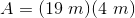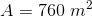# High School Math : How to find the area of a parallelogram

## Example Questions

### Example Question #7 : Parallelograms

What is the area of a parallelogram with a base ofand a height of?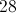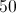Explanation:

To solve this question you must know the formula for the area of a parallelogram.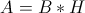In this equation,is the length of the base and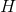is the length of the height. We can plug in the side length for both base and height, as given in the question.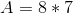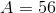### Example Question #8 : Parallelograms

What is the area of a parallelogram with a base ofand a height of?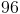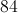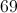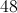Explanation:

To solve this question you must know the formula for the area of a parallelogram.

The formula isSo we can plug in the side length for both base and height to yield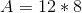Perform the multiplication to arrive at the answer of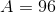.

### Example Question #9 : Parallelograms

Find the area of the following parallelogram: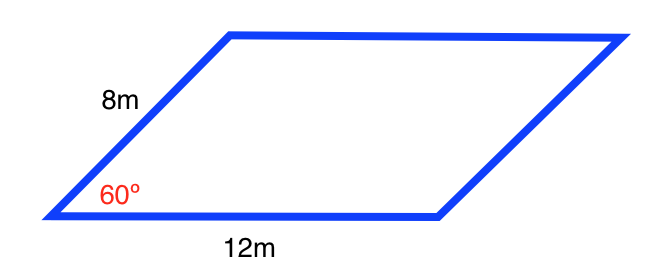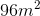Cannot be determined from the given information.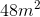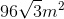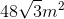Explanation:

The formula for the area of a parallelogram is: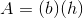,

whereis the length of the base andis the length of the height.

In order to the find the height of the parallelogram, use the formula for a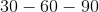triangle: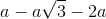, whereis the side opposite the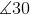.

The left side of the parallelogram forms the followingtriangle: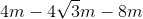, where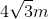is the length of the height.

Plugging in our values, we get: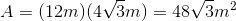### Example Question #10 : Parallelograms

Find the area of the following parallelogram: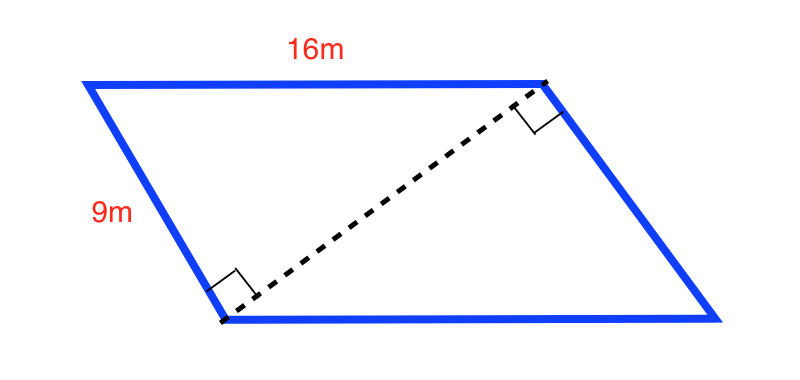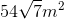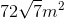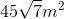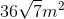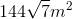Explanation:

Use the Pythagorean Theorem to determine the length of the diagonal: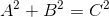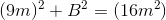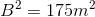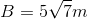The area of the parallelogram is twice the area of the right triangles: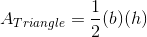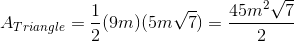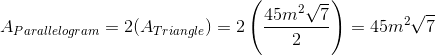### Example Question #31 : Quadrilaterals

Find the area of the following parallelogram: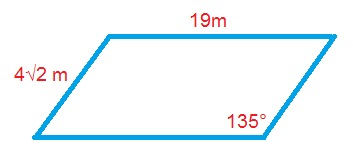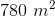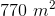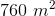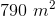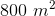Explanation:

The formula for the area of a parallelogram is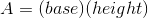.

Use the formula for a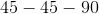triangle to find the length of the height: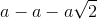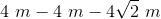Plugging in our values, we get: